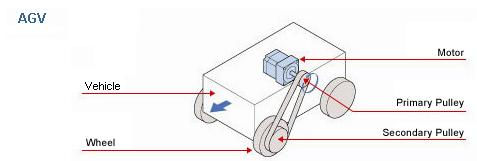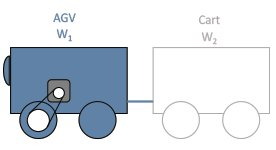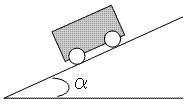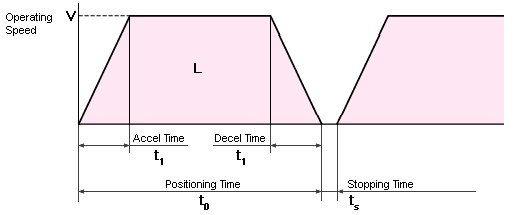Free Shipping for Online Orders. Terms and conditions apply.

## AGV - Automatic Guided Vehicle Sizing ToolSelect the unit

### Vehicle

Vehicle weight mass

W1 m1

= lb kg (Excluding the wheel weight mass)Wheel outer diameter

D1

= in mm

Wheel weight mass

WD1 mD1

= lb/pc kg/pc

Number of wheels

n1

= pcs

Rolling friction coefficient between wheel and floor

μ1

=

Reference:

Car tire on concrete: 0.010 to 0.015
Steel on steel: 0.02
Steel on wood: 0.22

Car tire on tar or asphalt: 0.030 to 0.035
Aluminum on steel: 0.10 to 0.15

Number of carts

N

= carts

### System efficiency

System efficiency

η

= %

### Transmission belt and pulleys or gears (Leave the fields blank if a direct coupling structure is used)

Primary pulley (gear) pitch circle diameter (PCD) or diameter

Secondary pulley (gear) pitch circle diameter (PCD) or diameter

Dp1

=   in mm

Dp2

=   in mm

Primary pulley (gear) weight mass

Secondary pulley (gear) weight mass

Wp1 mp1

=   lb kg

Wp2 mp2

=   lb kg

If you are not sure about the weight mass

If you are not sure about the weight mass

Primary pulley (gear) thickness

Secondary pulley (gear) thickness

Lp1

=   in mm

Lp2

=   in mm

Primary pulley (gear) material

Secondary pulley (gear) material

ρp1

=

ρp2

=

### Floor slope

Maximum angle of floor slope

α

= °### Other requirement(s)

It is necessary to hold the load even after the power supply is turned off.
→ You need an electromagnetic brake.

It is necessary to hold the load after the motor is stopped, but not necessary to hold after the power supply is turned off.

### Operating conditions

 Fixed speed operation Operating speed V1 = ft m /min Acceleration/Deceleration t1 = s
 Variable speed operation Operating speed V1 = ft/min m/min 〜 V2 = ft/min m/min Acceleration/Deceleration t1 = s
 Positioning operation (Fill in the fields, if any)Rotor inertia JO = oz·in kg·m 2 Gear ratio i = If the rotor inertia and the gear ratio are unknown, the acceleration torque will be calculated with an inertia ratio of 5:1 (see the motor selection tips that will appear on the result window for the detail).Positioning distance L = ft m Positioning time t0 = s Stopping time ts = s Acceleration / deceleration time is required t1 = s Operating speed V = ft/min m/min

### Stopping accuracy

Stopping accuracy

±

in mm

### Safety factor

Safety factor

The following is the estimated requirements. Please contact 1-800-468-3982 ( from overseas 1-847-871-5931 ) for assistance or questions.

### Sizing Results

JL

= [oz·in [kg·m 2]

Required Speed

Vm

= [r/min]

V2

= [r/min]

Required Torque

T

= [lb·in] = [oz·in] [N·m]

RMS Torque

Trms

= [lb·in] = [oz·in] [N·m]

Acceleration Torque

Ta

= [lb·in] = [oz·in] [N·m]

TL

= [lb·in] = [oz·in] [N·m]

Required Stopping Accuracy

Δθ

= [deg]

Other Requirement(s)

To print the calculation report, click    Full Report
To view the motor selection tips, click    Tips

×Call 1-800-GO-VEXTA(468-3982) or 1-847-871-5931Print

- given information -

### Vehicle

Vehicle weight mass

W1 m1

[lb] [kg]

Wheel outer diameter

D1

[in] [mm]

Wheel weight mass

WD1 mD1

[lb/pc] [kg/pc]

Number of wheels

n1

[pc]

Rolling friction coefficient between wheel and floor

μ1

=

Number of carts

N

carts

### System efficiency

Drive mechanism efficiency

η

%

### Transmission belt and pulleys or gears

Primary pulley (gear)

Secondary pulley (gear)

pitch circle diameter (PCD)

Dp1

= [in] [mm]

Dp2

= [in] [mm]

weight mass

Wp1 mp1

= [lb] [kg]

Wp2 mp2

= [lb] [kg]

thickness

Lp1

= [in] [mm]

Lp2

= [in] [mm]

material

ρp1

= [oz/in [kg/m 3]

ρp2

= [oz/in [kg/m 3]

### Floor slope

Maximum angle of floor slope

α

°

### Other requirement(s)

Is it necessary to hold the load even after the power supply is turned off?

Is it necessary to hold the load after the motor is stopped, but not necessary to hold after the power supply is turned off?

### Operating conditions

Fixed speed operation

Operating speed

V1

=

[ft/min] [m/min]

Acceleration / deceleration time

t1

=

[s]

### Operating conditions

Variable speed operation

Operating speed

V1

=

[ft/min] [m/min]

V2

=

[ft/min] [m/min]

Acceleration / deceleration time

t1

=

[s]

### Operating conditions

Positioning operation

Rotor inertia

JO

=

[oz·in kg·m 2]

Gear ratio

i

=

Positioning distance

L

=

[ft] [m]

Positioning time

t0

=

[s]

Stopping time

ts

=

[s]

Acceleration / deceleration time

t1

=

[s]

Specified speed

V

=

[ft/min] [m/min]

### Stopping accuracy

Stopping accuracy

Δl

= [in] [mm]

### Safety factor

Safety factor

S·F

=

- calculated result -

Vehicle

JV

=   (W1 + (1/2 ) WD1 × n1) × 16 × (D1 / 2)2 (m1 + (1/2 ) mD1 × n1) × (D1 × 10-3 / 2)2

=   ( + (1/2) × × ) × 16 × ( ×10-3  / 2)2

= [oz·in [kg·m 2]

Carts

JC

=   (W2 + (1/2 ) WD2 × n2) × 16 × (D1 / 2) (m2 + (1/2 ) mD2 × n2) × (D1 × 10-3 / 2) 2 × N

=   ( + (1/2) × × ) × 16 × ( ×10-3  / 2)2 ×

= [oz·in [kg·m 2]

Primary pulley

JDp1

=  ( 1 / 8 ) Wp1 × 16 × Dp1 mp1 × (Dp1×10-3) 2

=   ( 1 / 8 ) ×  × 16 × ( ×10-3) 2

= [oz·in [kg·m 2]

Primary pulley

JDp1

=   ( π / 32 ) ρp1 ( Lp1 ×10-3) ( Dp1 ×10-3) 4

=   ( 3.14 / 32 ) ×  × ( ×10-3)  × ( ×10-3) 4

= [oz·in [kg·m 2]

Secondary pulley

JDp2

=  ( π / 32 ) ρp2 ( Lp2 ×10-3) ( Dp2 ×10-3) 4

=   ( 3.14 / 32 ) ×  × ( ×10-3)  × ( ×10-3) 4

= [oz·in [kg·m 2]

Secondary pulley

JDp2

=   ( 1 / 8 ) Wp2 × 16 × Dp2 mP2 × (DP2×10-3) 2

=   ( 1 / 8 ) ×  × 16 × ( ×10-3) 2

= [oz·in [kg·m 2]

JL

=   ( JV + JC + JDp2 ) ( Dp1 / Dp2 )2 + JDp1

= (  +  +  ) × (  /  )2 +

[oz·in [kg·m 2]

JL

=   JV + JC

=  (  +  )

[oz·in [kg·m 2]

### Required Speed

 Vm =   ( 12 × V1) / ( π D1 × 10-3)   ( Dp2 / Dp1 ) =   (12 × ) / (3.14 × × 10-3) × (  /  ) = [r/min]
 Vm1 =   (12 × V1) / ( π D1 × 10-3 ) ( Dp2 / Dp1 ) (12 × ) / (3.14 × × 10-3) × (  /  ) = [r/min] Vm2 =   (12 × V2) / ( π D1 × 10-3) ( Dp2 / Dp1 ) (12 × ) / (3.14 × × 10-3) × (  /  ) = [r/min]
 Vm =   (12 × L) / ( π D1 × 10-3 ) × (60 / (t0 - t1)) ( Dp2 / Dp1 ) (12 × ) / (3.14 × × 10-3 ) × (60 / ( - ) ) × (  /  ) = [r/min] Vm =   (12 × V) / ( π D1 × 10-3 ) ( Dp2 / Dp1 ) (12 × ) / (3.14 × × 10-3) × (  /  ) = [r/min]

### Required Torque

T

=   ( Ta + TL ) ( Safety Factor )

= (  +  ) ×

= [lb·in] [N·m]

= [oz·in]

### RMS Torque

Trms

=

√(((( Ta + TL )2 × t1 ) + ( TL2 × (t0 - 2 × t1 )) + (( Ta - TL )2 × t1 )) / ( t0 + ts )) × (Safety Factor)

=

√ ((((  +  )2 ×  ) + ( 2 × (  - 2 ×  )) + ((  -  )2 ×  )) / (  +  )) ×

= [lb·in] [N·m]

= [oz·in]

### Acceleration Torque

 Ta = ( JL / 386 ) ( Vm / ( 9.55 × t1 )) ( 1 / 16 ) = (   / 386 ) × (  / ( 9.55 ×  )) × ( 1 / 16 ) = [lb·in] [N·m] = [oz·in]
 Ta = ( JL / 386 ) ( Vm / ( 9.55 × t1 )) ( 1 / 16 ) = (  / 386 ) × (  / ( 9.55 ×  )) × ( 1 / 16 ) = [lb·in] [N·m] = [oz·in]
 Ta = (( JO × i2 + 1.2 × JO + JL ) / 386 ) ( Vm / ( 9.55 × t1 )) ( 1 / 16 ) = ((  × 2 + 1.2 × +  )  / 386 )  × (   / ( 9.55 ×  ))  × ( 1 / 16 ) = [lb·in N·m] = [oz·in]

F

=   9.8 ( ( W m 1 + n1 × W m D1 ) × ( sinα + μ1 cosα ) + ( W m 2 + n2 × W m D2 ) × N × ( sinα + μ2 cosα ) )

=   9.8 ( ( + × ) × ( sin + × cos ) + ( + × ) × × ( sin + × cos ) )

[lb N]

TL

=   ( F × D1 ×10-3 ) / (2 η × 0.01 )   ( Dp1 / Dp2 )

=   (  ×   ×10-3 ) / ( 2 × × 0.01 )   × (  /  )

= [lb·in] [N·m]

=   [oz·in]

### Required Stopping Accuracy

Δθ

=  Δl ( 360° / π D1 ) ( Dp2 / Dp1 )

=    × ( 360 / (3.14 × )  ) × (  /  )

[deg]

### Other requirement(s)

- end of the report -